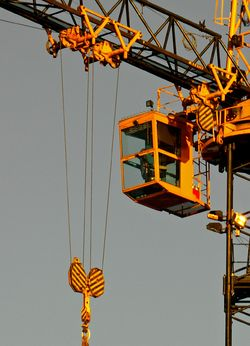# Simple machine

(diff) ← Older revision | Latest revision (diff) | Newer revision → (diff)Figure 1: Example of a simple machine, the pulley, in practical use.

A simple machine is a physical device that changes the magnitude or direction of a force. It should be noted that these machines do nothing to change the amount of energy used, just how hard it is to use that energy.

Machines in general are thought of as making human's lives easier, and simple machines are the foundation of any device of this nature. Such machines were defined in Renaissance days, and include levers, pulleys, inclined planes, wedges, the wheel and axle, gears and screws.

The underlying principle of a simple machine is the mechanical advantage that it provides. Mechanical advantage is the ratio of the output force of the machine to the force that was applied to it. This means that the applied force can be small, while the output force to an object can be much larger than it! This is limited by the conservation of energy however, , so although the output force can be large, the distance it travels must be smaller in direct proportion. The (ideal) mechanical advantage is the ratio of applied force to the output force: